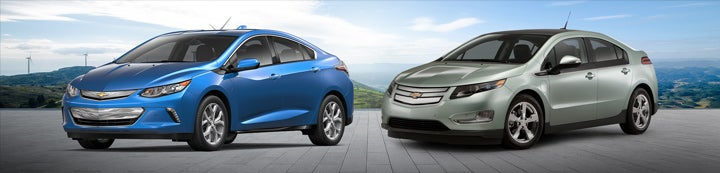1 - 5 of 5 Posts

George S. Bower

·
Registered
Joined
·
2,707 Posts
Discussion Starter · ·
The purpose of this analysis is to examine the Volt's planetary gear set. First, the equation describing the speed relationships between the sun, planet, and ring gear will be presented. Then the 70 MPH cruise condition with the power split clutch engaged will be examined. The power spilt between the ICE, generator, traction motor and the carrier will be derived.

The schematic for this condition is as follows:
fig1

Note that at this condition the Volt is requiring 27.3 HP to sustain a 70 MPH road speed.
Power is transmitted to the wheels thru a gearbox with a 2.16 gear ratio. This results in an output shaft (carrier speed from the PG set of 1930 RPM.

The next schematic shows the derivation of the PG set speed relationship:
fig2

We know that the gear ratio between the sun gear and the carrier with the ring gear locked is 3.24/1. We also know that the gear ratio between the ring gear and the carrier with the sun locked is 1.45/1. Using these ratios the equation for the speed relationship can be derived.

It is:

Nc=.31Nsun+.69 Nr

This equation simply implies that the torque at the output shaft is split in a ratio of 69% and 31% between the ring gear and the sun as shown below:
fig3

In order to figure the speed splits at this 70 MPH condition we need to pick an ICE speed which feeds into the ring gear of the PG set. I picked an ICE speed that results in the best SFC out of the ICE at a power of 27.3 HP (20.4 kw) which is the road load for this condition. We want to see if this will actually be the true match point at the end of this iteration.

The speed that gives the best SFC at 20 kw out of the ICE is 2300 RPM. That speed was chosen from a plot of HP versus engine speed for peak cycle efficiency. (I used a Prius engine map)

Using the selected speed of 2300 RPM for the ICE and 1930 RPM for the output (carrier) speed we can solve for the sun ( MGb) speed from the PG speed equation derived above. We also can split the torque using the 69%/31% torque split. Using those speeds and torques we can calculate the POWER SPLIT.

The resultant condition is shown below:
fig5

As you can see, The resultant power into the PG set almost exactly equal to the optimum power for best sfc out of the ICE at 27.3 HP road load. In this condition in order to get better SFC out of the ICE we need to add another 4.5 HP to the ICE.

We simply add 4.5 HP worth of generator load. So here we have a near perfect match between the ICE and the road load. 82% of the power generated from the ICE is being transmitted mechanically directly to the wheels. The remaining 18% power is being converted into electricity by the generator and then being fed to the main traction motor.

Conclusion:

Very efficient. The Volt has the potential for Prius MPG in CS Mode w/ power split engaged.

Rooster

·
Registered
Joined
·
706 Posts
fig5

As you can see, The resultant power into the PG set almost exactly equal to the optimum power for best sfc out of the ICE at 27.3 HP road load. In this condition in order to get better SFC out of the ICE we need to add another 4.5 HP to the ICE.

We simply add 4.5 HP worth of generator load. So here we have a near perfect match between the ICE and the road load. 82% of the power generated from the ICE is being transmitted mechanically directly to the wheels. The remaining 18% power is being converted into electricity by the generator and then being fed to the main traction motor.

Conclusion:

Very efficient. The Volt has the potential for Prius MPG in CS Mode w/ power split engaged.
Nice analysis George.

In this case, you are showing 4.5 HP out of M/G A, when M/G B requires 4.9 HP. So the difference would have to be dawn out of the battery, and would be greater than .4 HP due to conversion losses...assuming the average power required at the wheels is 27.3 HP

If M/G B requires approximately 5 HP on average, and M/G A is roughly 90% efficient providing it. M/G A will need to consume about 5.5 HP generating electricity, thus the ICE will need to provide more like 28 HP, right? Still pretty efficient.

ChrisC

·
Registered
Joined
·
1,208 Posts
One thing I'm not following is speed ratios versus torque ratios. Just because there's a 69%/31% shaft speed ratio at 70MPH in CS mode, doesn't mean that the torques applied are following that ratio. But it seems like you are saying that. Further the 22.5 / 4.9 / 27.3 torques in your last sketch don't follow the 69/31 relationship, so I think I'm missing something here. Sorry, I never took any gear mechanics classes, and even if I did it was a very long time agoGeorge S. Bower

·
Registered
Joined
·
2,707 Posts
Discussion Starter · ·
One thing I'm not following is speed ratios versus torque ratios. Just because there's a 69%/31% shaft speed ratio at 70MPH in CS mode,
The 69/31 ratio is the torque split--- which is constant. Just the speeds change and therefore the power.

whistles28

·
Registered
Joined
·
2 Posts
Hi, has anyone figured out the real sfc curves of the VOLT? I surely would like to know that information!...

1 - 5 of 5 Posts